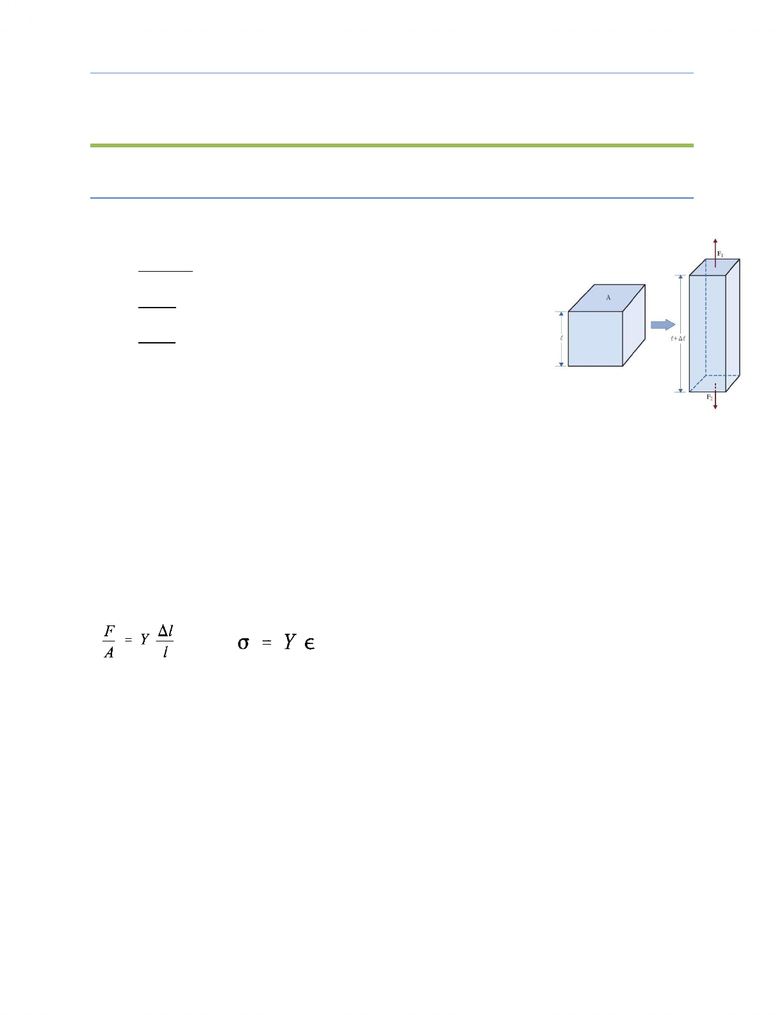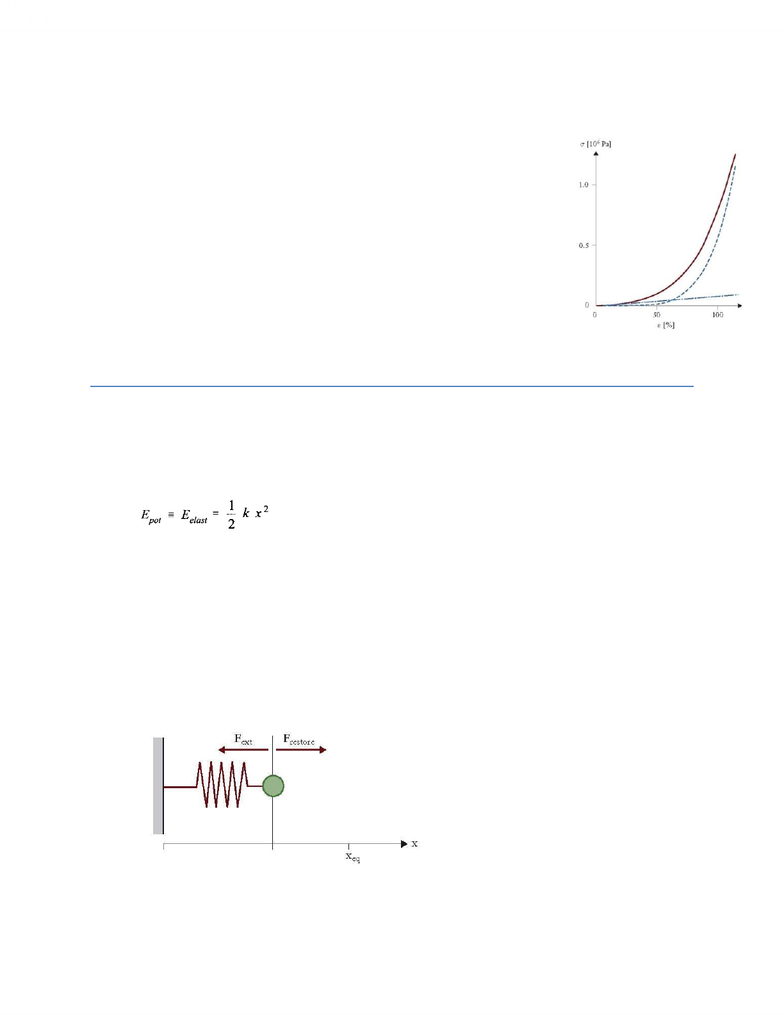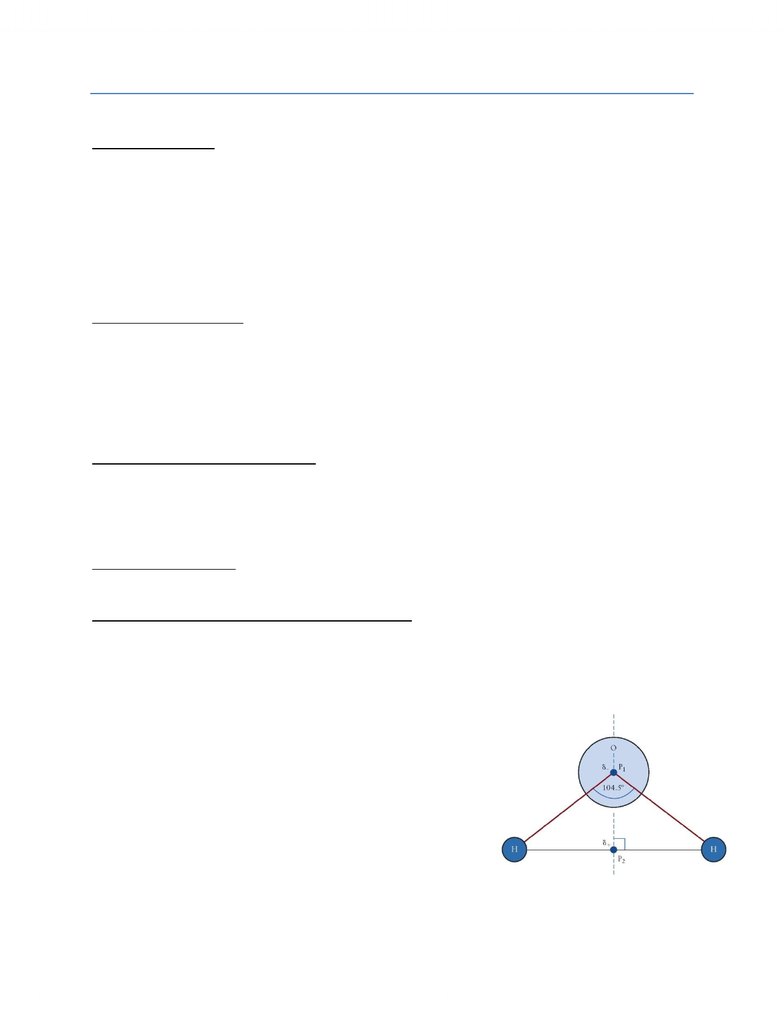# Physics 1021 Study Guide - Final Guide: Elastic Energy, Electric Dipole Moment, Electric Potential Energy

68 views10 pages
School
Western University
Department
Physics
Course
Physics 1021Conceptual Physics - Final Exam Notes
Elastic Materials Pg. 496 509
What is an elastic body?
Responds with change of shape to external forces
Elasticity: tensile stress and stretching
The response to stretching is stress and strain
Stress: a force exerted per unit area of surface of the extended
object, has unit of [Pa]
Strain: relative change of length or size of the object; three types:
o Stretching is a change in the length of the object
o Twisting is a change in an angle of the extended object
o Compression or expansion is a change in the volume of an
object
Each type of strain is caused by a different form of stress
o Stretching is caused by tensile stress.
o Twisting is caused by shearing stress.
o Compression or expansion is caused by hydraulic stress.
Elastic Deformation
Stress will cause deformation of an object. If, after the stress is removed, the object returns to its
original shape, the deformation was elastic. Stress and strain are proportional to each other in elastic
formation.
Linear Response (Hooke’s Law)
If stress and strain are linearly proportional to each other, we can write:
OR
Near mechanical equilibrium, a linear relation exists between the cause of the deformation
(stress) and the response by the extended object (strain)
The linear relation between stress and strain is called Hooke’s law.
The proportionality factor Y is called Young’s modulus with a unit of [Pa]
Large Y means that a large force acting on a material leads only to a small length variation.
Materials with a large Y are called strong (hard) materials. Examples are steel and your desk.
Small Y means that a large force acting on a material causes a large length variation. Materials
with small Y are called soft materials. Blood vessels and skin are examples of soft materials.
When the response is linear, it is also elastic.
Elastic vs. Plastic Deformations
A material is called elastic when it responds to external forces (stress) with a linear deformation
(strain). Elastic deformations are reversible (the object resumes its original shape when the
stress is removed).
Unlock document

This preview shows pages 1-3 of the document.
Unlock all 10 pages and 3 million more documents.A material is called plastic when it responds to a stress in a non-linear fashion. Plastic
deformations are irreversible (the strain does not return to zero when the stress on the material
is removed).
Elastic Tissue of Blood Vessels
Blood vessels are non-elastic
Combined effect of collagen and elastin (structural protein) result in
elasticity of blood vessels
Not included: contractile smooth muscles
Collagen keeps the blood vessel intact (it is due to collagen that the actual
blood vessel walls do not show linear stress-strain behaviour, as indicated
by the diagram)
Diagram: collagen blue dashed; elastin dot dash; solid line combined
effect
The elastic force is also called a restoring force.
Vibrations Pg.509 516
Elastic Potential Energy
kx = m(d²x/dt²)
Elastic potential energy is the energy that depends on the displacement of the object.
A system with a linear restoring force (Hooke’s law) has an elastic potential energy that is
proportional to the square of the displacement from the equilibrium position of the system.
Elastic potential energy is not linear in the displacement of the object; elastic potential energy
plots are not shown as straight lines are gravitational potential energy plots are, but as curves,
where energy is conserved.
Thus, when the displacement from the equilibrium position doubles, the elastic energy increases
by a factor of 4.
The vibration of an object attached to a spring
o For a small displacement Δ x = x xeq the external force is linearly proportional to the
displacement, Δ x from the equilibrium position. (Hooke’s law)
o Frestore = Felast is the force exerted on the object by the spring. The object is at equilibrium.
o Diagram:
Observations made from the spring model
The amplitude of the motion of an object attached to a spring is the maximum displacement
of a vibrating object.
Simple harmonic motion (when an object oscillates about a point of mechanical equilibrium)
and angular frequency
Unlock document

This preview shows pages 1-3 of the document.
Unlock all 10 pages and 3 million more documents.Electric Force Pg. 404 413, 419 420
Properties of Water
Large surface tension
The water molecule consists of two hydrogen atoms and one oxygen atom connected by covalent bonds
(H2O) and arranged at an angle of 104.5. The oxygen end of the molecule carries a partial negative
charge and the hydrogen end a partial positive charge. Opposite charges separated by a fixed angle
define a dipole.
Water molecules stick to one another as a result of hydrogen bonding; even though hydrogen
bonds are weak they hold water strongly at a macroscopic level. This is known as cohesion.
Surface tension is the related measure of how much effort is needed to increase the surface
area of a liquid.
High specific heat capacity
The amount of energy required for the phase transition of a material from the liquid to the
vapour state at its boiling point.
Hydrogen bonds have a large latent heat vaporization because they need to be broken during
evaporation.
A large amount of energy is required when water evaporates from a surface.
This effects climate and the heat absorbed by evaporation in oceans.
Ice floats on the surface of liquid water
ρ ice > ρ liquid water
Water is less dense in solid form than in liquid form
Most materials contract when they solidify but water expands
Floating ice thermally insulates the water below
Boiling and melting point
Water has a high boiling and melting point
Stabilizes salts and polar organic molecules in a solution
Effective solvent in chemistry
Water molecules form a hydration shell large number of molecules that form a layer around a
charged particle that stabilizes the ions in the solution
The hydration shell is energetically favoured because of an electric interaction between the
charged particle and the water molecule
All of these features are due to the one microscopic feature of the
water molecule water is an electric dipole.
Opposite charges separated by a fixed angle define a dipole.
Dipoles interact electrically with ions and other dipoles.
The positive end of one dipole and negative end of another
attract each other in liquid water.
Water dipoles form hydrogen bridge bonds.
Electric Charge and Force
Electric charge is an intrinsic property of the particles that comprise matter in the same fashion
as mass is an intrinsic property of the same particles. Because particles carrying single charges
are usually very small, the concept of point charge is introduced. A point charge is a charged
particle with negligible (insignificant) size.
Unlock document

This preview shows pages 1-3 of the document.
Unlock all 10 pages and 3 million more documents.

## Document Summary

Responds with change of shape to external forces. The response to stretching is stress and strain. Stress: a force exerted per unit area of surface of the extended object, has unit of [pa] Each type of strain is caused by a different form of stress: stretching is caused by tensile stress, twisting is caused by shearing stress, compression or expansion is caused by hydraulic stress. If, after the stress is removed, the object returns to its original shape, the deformation was elastic. Stress and strain are proportional to each other in elastic formation. If stress and strain are linearly proportional to each other, we can write: Near mechanical equilibrium, a linear relation exists between the cause of the deformation (stress) and the response by the extended object (strain) The linear relation between stress and strain is called hooke"s law. The proportionality factor y is called young"s modulus with a unit of [pa]